Latest Banking jobs   »

# Reasoning Ability Quiz For LIC ADO Prelims 2023- 11th March

Directions (1-3): Study the information carefully and answer the questions given below.
Seven persons A, B, C, D, E, F and G are sitting in a circular table. Some of them facing towards center and some of them facing away from center but not necessary in same order.
D sits third to the right of B. E sits immediate left of D. Two persons sit between B and G. A is immediate neighbor of C. A sits third to the right of F. Immediate neighbor of G face opposite direction to each other. G face towards the center. Neither G nor C face same direction as D. Not more than four-person face towards center.

Q1. Who among the following person sits second to the right of C?
(a) F
(b) D
(c) E
(d) G
(e) None of these

Q2. How many persons sit between A and E, when counted to the right of E?
(a) Two
(b) Three
(c) One
(d) Four
(e) None of these

Q3. How many persons face outside the center?
(a) Four
(b) Two
(c) Three
(d) Five
(e) None of these

Q4. If all the digits in the number “8154276367” arranged in ascending order from left to right then how many numbers remains same in their position?
(a) One
(b) Two
(c) Three
(d) Four
(e) None of these

Q5. If ‘A × D’ mean ‘D is the sister of A’, ‘A + D’ means ‘D is the daughter of A’, ‘A ÷ D’ means ‘A is the wife of D’, and ‘A-D’ means ‘A is brother of D’ then how will ‘K is mother of P’ be denoted?
(a) J – K ÷ P + L
(b) K + P + M × J
(c) J – K ÷ L + P
(d) K – J ÷ L + P
(e) None of these

Directions (6-10): In each of the questions below some statements are given followed by some Conclusions. You have to take the given statements to be true even, if they seem to be at variance from commonly known facts. Read all the conclusions and then decide which of the given conclusions logically follows from the given statements disregarding commonly known facts.
(a) If only conclusion I follows.
(b) If only conclusion II follows.
(c) If either conclusion I or II follows.
(d) If neither conclusion I nor II follows.
(e) If both conclusions I and II follow.

Q6. Statements: Some Apple are Mango
All Mango are Sound
No sound is Travel
Conclusion: I: Some apple are not travel
II: No mango are apple

Q7. Statements: All room are wood
Some core are room
Some Test are Room
Conclusion: I: All Test can be wood
II: Some core are wood

Q8. Statements: Some Left are right
All right are true
All true are wrong
Conclusions: I. All left are wrong
II. Some left are not wrong

Q9. Statements: Some words are magic.
Some magic is Heart.
All Heart are Music.
Conclusions: I. Some words are Music
II. No word is music

Q10. Statements: Some dam are River
Some River are Gold
No River is Silver
Conclusions: I: All Dam can be Gold
II: Some Gold are not Silver

Q11. How many such numerals are there in the number ‘4185476429’ which will remain at the same position when arranged in ascending order from left to right?
(a) None
(b) Three
(c) More than three
(d) Two
(e) None of these

Direction (12-15): In each of the questions below some statements are given followed by some conclusions. You have to take the given statements to be true even if they seem to be at variance with commonly known facts. Read all the conclusions and then decide which of the given conclusions logically follows from the given statements disregarding commonly known facts.
(a) If only conclusion I follows.
(b) If only conclusion II follows.
(c) If either conclusion I or II follows.
(d) If neither conclusion I nor II follows.
(e) If both conclusions I and II follow.

Q12. Statements: Only a few Bubbles are Soap.
All Soap are Margo.
Some Neem are not Margo.
Conclusions: I. Some Bubbles are not Neem.
II. Some Soap are not Bubbles.

Q13. Statements: All Ship are Jeep.
All Jeep are Bike.
Some Car are Bike.
Conclusions: I. Some Ship can be Car.
II. All Car are Bike.

Q14. Statements: Some Egg are Roll.
Some Roll are not Paratha.
Conclusions: I. All Egg can never be Paratha.
II. Some Paratha are not Roll.

Q15. Statements: All Noun are Pronoun.
Some Pronoun are Verb.
Some Verb are Tense.
Conclusions: I. Some Noun can be Verb.
II. Some Tense being Pronoun is a possibility.

Solutions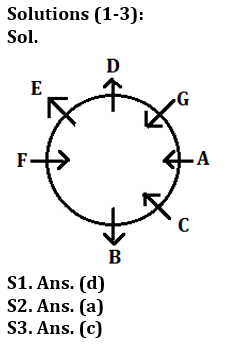S4. Ans. (b)
Sol. Original number: 8154276367
Obtained number: 1234566778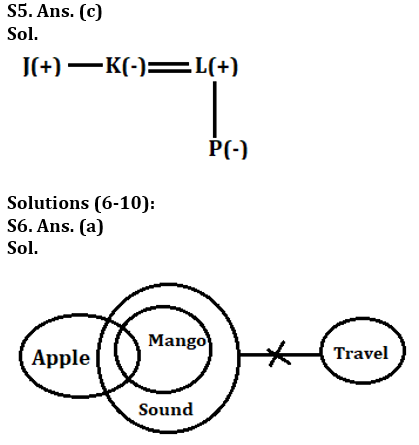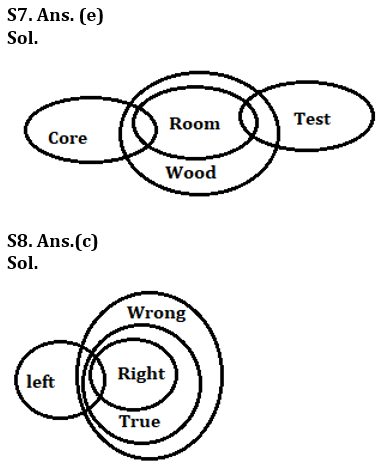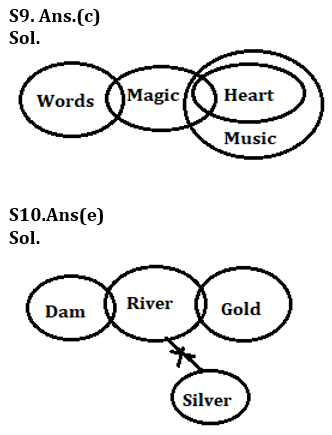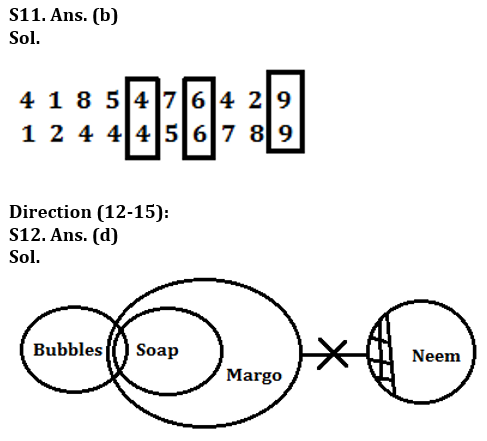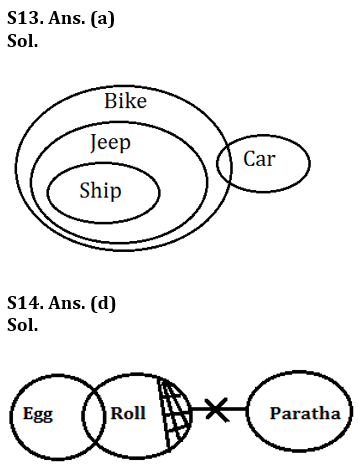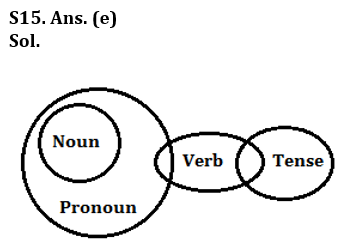## FAQs

### When will the LIC AAO Prelims Exam 2023 be conducted?

The LIC AAO Prelims Exam 2023 will be conducted on 17th & 20th February 2023.

#### Congratulations!Union Budget 2023-24: Free PDF